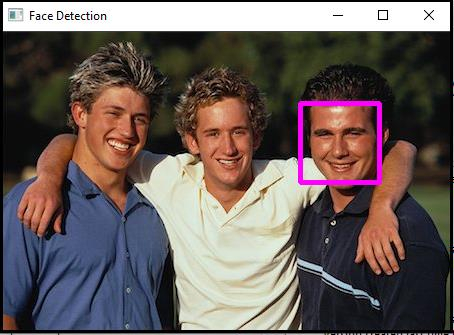# How to detect the largest face in OpenCV using C++?

OpenCVC++Server Side ProgrammingProgramming

We will learn how to detect the largest face only. This topic is same as the previous topic. The only difference is we used an additional 'Rect' structure and a 'for loop' to detect the largest face.

The actual format of this function −

Mat faceROI = image_with_humanface(maxRect)

The maxRect have the area and location information of the largest face located on the image. The line above is cropping the same area stored in maxRect on the same location where the largest face is located on the image and storing in 'faceROI' matrix.

The following program detects the largest face from still pictures −

## Example

#include<iostream>
#include<opencv2/highgui/highgui.hpp>
#include<opencv2/imgproc/imgproc.hpp>
//This header includes definition of 'rectangle()' function//
#include<opencv2/objdetect/objdetect.hpp>
#include<string>
using namespace std;
using namespace cv;
int main(int argc, char** argv) {
Mat image_with_humanface;//Declaring a matrix to load image with human faces//
namedWindow("Face Detection");//Declaring an window to show the result//
string trained_classifier_location = "C:/opencv/sources/data/haarcascades/haarcascade_frontalface_alt.xml";//Defining the location our XML Trained Classifier in a string//
vector<Rect>faces;//Declaring a rectangular vector named faces//
faceDetector.detectMultiScale(image_with_humanface, faces, 1.1, 4, CASCADE_FIND_BIGGEST_OBJECT);//Detecting the faces in 'image_with_humanfaces' matrix//
Rect maxRect;//Declaring a rectangle. By default the size is zero pixel//
for (int i = 0; i < faces.size(); i++){ //Initiating for loop to detect the largest face//
if (faces[i].area() > maxRect.area()){ //Calculating the area of face and comparing it with area of maxRect//
maxRect = faces[i];//storing the largest rectangle in MaxRect according to size of largest face//
}
}
for (size_t i = 0; i < faces.size(); i++){ //Loop to draw rectangle around the faces//
Mat faceROI = image_with_humanface(maxRect);//Storing the face in a matrix having size equal to maxRect//
int x = maxRect.x;//Getting the initial row value of face rectangle's starting point//
int y = maxRect.y;//Getting the initial column value of face rectangle's starting point//
int h = y + maxRect.height;//Calculating the height of the rectangle//
int w = x + maxRect.width;//Calculating the width of the rectangle//
rectangle(image_with_humanface, Point(x, y), Point(w, h), Scalar(255, 0, 255), 3, 6, 0);//Drawing a rectangle using around the largest//
}
imshow("Face Detection",image_with_humanface);//Showing the largest face face//
waitKey(0);//To wait for keystroke to terminate the program
return 0;
}

## Output